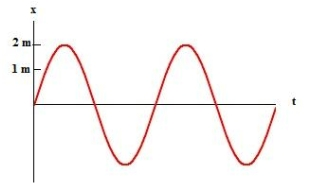Try out our new practice tests completely free!# Fundamentals of Physics Study Set 2

Physics & Astronomy

## Quiz 15 : OscillationsStudy FlashcardsLooking for Introductory Physics Homework Help?

## Quiz 15 :OscillationsA particle is in simple harmonic motion with period T.At time t=0 it is halfway between the equilibrium point and an end point of its motion, travelling toward the end point.The next time it is at the same place is:
Free
Multiple Choice

C

Tags
Choose question tagAn object attached to one end of a spring makes 20 vibrations in 10 seconds.Its frequency is:
Free
Multiple Choice

A

Tags
Choose question tagThe amplitude and phase constant of an oscillator are determined by:
Free
Multiple Choice

E

Tags
Choose question tagThis plot shows a mass oscillating as x = xm cos (ωt + φ).What are xm and φ?Multiple Choice
Tags
Choose question tagTwo identical undamped oscillators have the same amplitude of oscillation only if:
Multiple Choice
Tags
Choose question tagWhen a body executes simple harmonic motion, its acceleration at the ends of its path must be:
Multiple Choice
Tags
Choose question tagAn object attached to one end of a spring makes 20 complete vibrations in 10s.Its period is:
Multiple Choice
Tags
Choose question tagThe amplitude of any oscillator will be doubled by:
Multiple Choice
Tags
Choose question tagAn oscillatory motion must be simple harmonic if:
Multiple Choice
Tags
Choose question tagIt is impossible for two particles, each executing simple harmonic motion, to remain in phase with each other if they have different:
Multiple Choice
Tags
Choose question tagA particle moves back and forth along the x axis from x = -xm to x = +xm, in simple harmonic motion with period T.At time t = 0 it is at x = +xm.When t = 0.75T:
Multiple Choice
Tags
Choose question tagA block attached to a spring oscillates in simple harmonic motion along the x axis.The limits of its motion are x = 10 cm and x = 50 cm and it goes from one of these extremes to the other in 0.25 s.Its amplitude and frequency are:
Multiple Choice
Tags
Choose question tagA particle moves in simple harmonic motion according to x = 2cos(50t), where x is in meters and t is in seconds.Its maximum velocity is:
Multiple Choice
Tags
Choose question tagFrequency f and angular frequency are related by
Multiple Choice
Tags
Choose question tagA particle is in simple harmonic motion with period T.At time t = 0 it is at the equilibrium point.At the times listed below it is at various points in its cycle.Which of them is farthest away from the equilibrium point?
Multiple Choice
Tags
Choose question tagIn simple harmonic motion, the displacement is maximum when the:
Multiple Choice
Tags
Choose question tagA weight suspended from an ideal spring oscillates up and down with a period T.If the amplitude of the oscillation is doubled, the period will be:
Multiple Choice
Tags
Choose question tagAn object is undergoing simple harmonic motion.Throughout a complete cycle it:
Multiple Choice
Tags
Choose question tagA particle oscillating in simple harmonic motion is:
Multiple Choice